Practice the AP 9th Class Physical Science Bits with Answers Chapter 5 What is inside the Atom? on a regular basis so that you can attempt exams with utmost confidence.

## AP State Syllabus 9th Class Physical Science Bits 5th Lesson What is inside the Atom? with Answers

Question 1.
An atom is electrically
A) positive
B) negative
C) neutral
D) no change
C) neutral

Question 2.
The smaller particles present inside the atom are called
A) elements
B) protons
C) electrons
D) sub-atomic particles
D) sub-atomic particlesQuestion 3.
The important sub-atomic particles inside the atom are
A) protons
B) neutrons
C) electrons
D) all the above
D) all the above

Question 4.
The negatively charged particles are
A) electrons
B) protons
C) neutrons
D) none
A) electrons

Question 5.
The positively charged particles are
A) electrons
B) protons
C) neutrons
D) none
B) protons

Question 6.
The chargeless particles are
A) electrons
B) protons
C) neutrons
D) none
C) neutrons

Question 7.
The charge of alpha particles is
A) positive
B) negative
C) neutral
D) none
A) positive

Question 8.
Rutherford’s model is known as
A) plum pudding model
B) nuclear model
C) watermelon model
D) positive model
B) nuclear model

Question 9.
The nucleons are
A) protons and electrons
B) electrons and neutrons
C) protons and neutrons
D) neutrons
C) protons and neutronsQuestion 10.
Rutherford’s model could not explain
A) positivity of the atom
B) negativity of the atom
C) neutrality of the atom
D) stability of the atom
D) stability of the atom

Question 11.
According to Neils Bohr’s model, electrons are present in
A) shells
B) nucleus
C) outside the atom
A) shells

Question 12.
Most of the mass of an atom is concentrated in
A) protons
B) electrons
C) neutrons
D) nucleus
D) nucleus

Question 13.
n = 2, represents ………………. shell.
A) K
B) L
C) M
D) N
B) L

Question 14.
Maximum number of electrons that can be accommodate in a shell is given by the formula
A) 2n
B) n2
C) 2n2
D) 2n3
C) 2n2

Question 15.
Maximum number of electrons that can be accommodated in N shell is
A) 2
B) 32
C) 16
D) 18
B) 32

Question 16.
The valency of sulphur is
A) 2
B) 6
C) 2 and 6
D) 0
C) 2 and 6Question 17.
The valency of neon is
A) 1
B) 3
C) 2
D) 0
D) 0

Question 18.
In im1 Al the number of neutrons are
A) 14
B) 13
C) 27
D) 40
A) 14

Question 19.
The number of nucleons is the
A) atomic number
B) mass number
C) number of neutrons
D) number of protons
B) mass number

Question 20.
The …………………. isotope is used in the treatment of goitre.
A) isotope of iodine
B) isotope of uranium
C) isotope of cobalt
D) isotope of hydrogen
A) isotope of iodine

Question 21.
Which of the following is an example of isotopes ?
A) $${ }_{1}^{1} \mathrm{H},{ }_{1}^{2} \mathrm{H},{ }_{1}^{3} \mathrm{H}$$
B) $${ }_{6}^{12} \mathrm{C},{ }_{6}^{13} \mathrm{C},{ }_{6}^{14} \mathrm{C}$$
C) $${ }_{17}^{35} \mathrm{Cl},{ }_{17}^{37} \mathrm{Cl}$$
D) All the above
D) All the above

Question 22.
The mass of neutron is nearly equal to that of ………………….
A) proton
B) electron
C) α – particle
D) β – particle
A) proton

Question 23.
According to Thomson, the total ………………… of the atom is uniformly distributed throughout the atom.
A) volume
B) density
C) pressure
D) mass
D) massQuestion 24.
Rutherford allowed the alpha particles to pass through ……………………. in his
alpha particles scattering experiment.
A) aluminium foil
B) silver foil
C) copper foil
D) gold foil
D) gold foil

Question 25.
Alpha particles do not have …………………
A) electrons
B) protons
C) neutrons
D) none of these
A) electrons

Question 26.
Statement – I: Alpha particles consists of 2 protons.
Statement – II : Alpha particles con-sists of 4 neutrons.
A) Both the statements are true.
B) Statement -1 true and Statement – II false.
C) Statement -1 false and Statement – II true.
D) Both the statements are false.
A) Both the statements are true.

Question 27.
According to Bohr’s model, electrons revolve around nucleus in …………………
A) orbits
B) shells
C) orbitals
D) both A & B
D) both A & B

Question 28.
If n = 3, it represents the ……………….. shell.
A) K
B) L
C) M
D) N
C) M

Question 29.
According to Rutherford, the mass of the atom is concentrated at ………………..
A) orbit
B) shell
C) orbital
D) nucleus
D) nucleus

Question 30.
Electrons must ……………… energy to move to a higher energy level.
A) absorb
B) emit
D) no change
A) absorbQuestion 31.
Electrons must ………………. energy to move to a lower energy level.
A) absorb
B) emit
D) both B & C
D) both B & C

Question 32.
According to Bohr, while electrons are revolving in …………………..
A) discrete
B) stationary
C) higher energy
D) both A & B
D) both A & B

Question 33.
Bohr’s model could not explain the spectra of ……………….
A) Hydrogen
B) He+
C) Li2+
D) Heavier atoms
D) Heavier atoms

Question 34.
The rules for distribution of electrons is given by …………………
A) Bohr
B) Rutherford
C) Bury
D) Both A & C
D) Both A & C

Question 35.
Maximum number of electrons that can be accommodated in M – shell is ………………
A) 2
B) 8
C) 18
D) 32
C) 18

Question 36.
The electronic structure of oxygen is ………………
A) 2, 4
B) 2, 6
C) 2, 8
D) 2, 8, 2
B) 2, 6

Question 37.
The number of electrons present in outer most orbit of an atom is called …………………… electrons.
A) valency
B) paired
C) unpaired
D) love pair
A) valencyQuestion 38.
An outer most shell which has 8 elec-trons is said to possess an ………………. configuration.
A) dublet
B) octet
C) triplet
D) tetralet
B) octet

Question 39.
An atom with 8 electrons in its outer most shell is chemically …………………
A) stable
B) unstable
C) both
D) none of these
A) stable

Question 40.
Atoms of an element react with other atoms, to achieve ………………….. in their outer most shell.
A) singlet
B) octet
C) triplet
D) tetralet
B) octet

Question 41.
An atom can achieve octet by …………………. or of electrons.
A) transfer
B) sharing
C) both A and B
D) none of these
C) both A and B

Question 42.
Transfer or sharing of electrons by an atom results in the formation of ……………………. between the atoms.
A) attractive forces
B) chemical bond
C) repulsive force
D) both A & B
C) repulsive force

Question 43.
The number of protons in the nucleus of an atom is its …………………
A) atomic number
B) mass number
C) atomic mass
D) atomic volume
A) atomic number

Question 44.
The number of nucleons is called ……………….. of the atom.
A) atomic number
B) atomic mass number
C) atomic mass
D) atomic volume
B) atomic mass numberQuestion 45.
Number of neutrons N = ……………….
A) A – Z
B) A + Z
C) A × Z
D) A / Z
A) A – Z

Question 46.
Atomic number is denoted by
A) A
B) Z
C) A – Z
D) A + Z
B) Z

Question 47.
Mass number is denoted by
A) Z
B) A – Z
C) A
D) A + Z
C) A

Question 48.
The elements which have a highest number of known isotopes ……………….. are and ……………..
A) Xenon, Cesium
B) Sodium, Potassium
C) Calcium, Strontium
A) Xenon, Cesium

Question 49.
The isotopes of Uranium is used as fuel in ………………. reactors.
A) Thermal
B) Hydro
C) Wind
D) Nuclear
D) Nuclear

Question 50.
The isotope of …………………. is used in treatment of cancer.
A) Iron
B) Sodium
C) Iodine
D) Cobalt
D) Cobalt

Question 51.
$${ }_{1}^{2} \mathrm{H}$$is called …………………
A) Hydrogen
B) Deuterium
C) Tritium
D) None of these
B) DeuteriumQuestion 52.
Xenon and cesiums have …………….. known isotopes.
A) 30
B) 32
C) 36
D) 40
C) 36

Question 53.
……………….. are not part of nucleus.
A) Protons
B) Electrons
C) Positrons
D) Neutrons
B) Electrons

Question 54.
Electron configuration of Neon is ………………..
A) 2
B) 2, 8
C) 2, 8, 8
D) 2, 8, 7
B) 2, 8

Question 55.
Match the following.

 a) Carbon 1) 2, 8, 8 b) Argon 2) 2, 8, 7 c) Chlorine 3) 2 d) Helium 4) 2, 4

A) a → 4, b → 1, c → 2, d → 3
B) a→ 3, b → 2, c → 1, d → 4
C) a → 2, b → 3, c → 4, d → 1
D) a → 1, b → 2, c → 3, d → 4
A) a → 4, b → 1, c → 2, d → 3

Question 56.
Match the following.

 a) Proton 1) e– b) Electron 2) n0 c) Neutron 3) p+

A) a → 2, b → 1, c → 3
B) a → 3, b → 1, c → 2
C) a → 1, b → 2, c → 3
D) a → 2, b → 3, c → 1
B) a → 3, b → 1, c → 2

Question 57.
Which of the following is a wrong statement ?
A) Mass of proton was 1836 times greater than that of the electron
B) Proton is represented as P
C) Proton repels neutrons
D) Proton is a subatomic particle in an atom
B) Proton is represented as P

Question 58.
If there are no protons in an atom, the consequences are
A) all atoms possess negative charge.
B) all atoms possess positive charge.
C) all atoms possess neutral charge.
D) above all
A) all atoms possess negative charge.Question 59.
‘X’ is a subatomic particle. It has neither +ve nor -ve. Then ‘X’ is
A) proton
B) positron
C) electron
D) neutron
D) neutron

Question 60.
If an α – particle comes closure to a proton, it …………………. proton.
A) attracts
B) repels
C) no change
D) first attracts and then repels
B) repels

Question 61.
Electron do not fall in nucleus, because
A) electron do not radiate energy while rotating in discrete orbits.
B) electron radiates energy while rotating in discrete orbits.
C) the size of nucleus is very small to attract the electron.
D) A and C
A) electron do not radiate energy while rotating in discrete orbits.

Question 62.
Fluorine atom contains seven electrons in outermost shell, but its valency is ‘1’. Predict the reason.
A) It can loss six electrons from the valency shell.
B) It can loss only one electron from the valency shell.
C) It can gain only one electron.
D) It can gain only seven electrons.
B) It can loss only one electron from the valency shell.

Question 63.
According to the electrolysis experiments conducted by …………….., the atoms were acquiring negative charge during the process of electrolysis.
A) Dalta
C) Rutherford
D) Bohr

Question 64.
Mass of electron is …………….. times less than that of proton.
A) 1200
B) 1836
C) 1830
D) 1870
B) 1836

Question 65.
Neutrons were discovered by …………………
A) J.J. Thomson
B) Rutherford
C) Goldstein

Question 66.
The atoms of same element which have same numbers of protons, but have different number of neutrons are called ………………….
A) Isobars
B) Isotopes
C) Isotones
D) Isomers
B) IsotopesQuestion 67.
Match the following.

 a) Plum pudding or watermelon model of atom 1) Goldstein b) Proton 2) J.J. Thomson c) Sodium 3) 2, 8, 1

A) a → 3, b → 1, c → 2
B) a → 2, b → 1, c → 3
C) a→ 1, b → 2, c → 3
D) a → 3, b → 2, c → 1
B) a → 2, b → 1, c → 3

Question 68.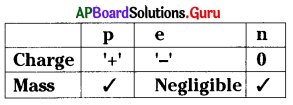From the table, which of the following is not correct ?
A) Electron has negative charge
B) Neutron has no charge
C) Proton has both mass and charge
D) Mass of the electron is very very less
B) Neutron has no charge

Question 69.
Rutherford : ………………. :: JJ Thomson : Watermelon
A) planetary system
B) coconut
C) α – particle
D) big bang
A) planetary system

Question 70.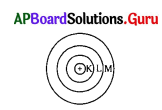In the figure …………………. has more energy.
A) K – shell
B) L – shell
C) M – shell
D) All are equal
C) M – shell

Question 71.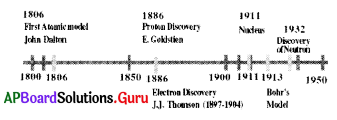From the given time line, which of the sub-atomic particle discovered at last?
A) proton
B) electron
C) neutron
D) none
B) electron

Question 72.
The given atom is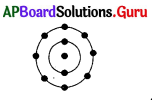A) He
B) O
C) Ne
D) Ar
C) Ne

Question 73.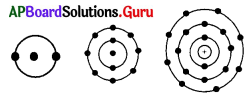The common property of the above atoms is
A) same number of shells
B) same atomic number
C) same valency
D) above all
C) same valencyQuestion 74.
Electrons distribution in the given diagram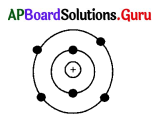A) 2, 6
B) 2, 4
C) 2, 2
D) 0, 8
B) 2, 4

Question 75.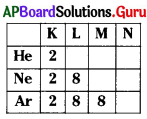The outermost orbit in ‘Ne’ is
A) K
B) L
C) M
D) N
B) L

Question 76.
From the above table no. of protons in ‘Ar’ is
A) 8
B) 16
C) 18
D) 10
C) 18

Question 77.
From the above table valency of ‘Ar’ is
A) 8
B) 2
C) 18
D) 0
D) 0

Question 78.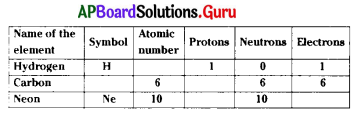What is the symbol of carbon ?
A) Ca
B) C
C) Cr
D) Cl
B) C

Question 79.
From the above table, what is the atomic number of hydrogen ?
A) 1
B) 2
C) 3
D) 0
A) 1

Question 80.
From the above table no. of protons Neon is
A) 5
B) 4
C) 6
D) 10
D) 10Question 81.
Which of the sub-atomic particle is not in the hydrogen?
A) proton
B) electron
C) neutron
D) none
C) neutron

Question 82.
$${ }_{6}^{14} \mathrm{C},{ }_{6}^{13} \mathrm{C},{ }_{6}^{12} \mathrm{C}$$ what are these ?
A) Isotopes
B) Isobars
C) Isotones
D) None
A) Isotopes

Question 83.
In $${ }_{6}^{14} \mathrm{C}$$, how many neutrons are there ?
A) 6
B) 14
C) 8
D) 20
C) 8

Question 84.
Below experiment done by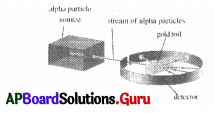A) Bohr
B) Thomson
C) Rutherford
D) Hund’s
C) Rutherford

Question 85.
Correct labelling of the given diagram is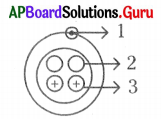A) 1 – proton, 2 – neutron, 3 – electron
B) 1 – neutron, 2 – proton, 3 – electron
C) 1 – electron, 2 – neutron, 3 – proton
D) 1 – electron, 2 – proton, 3 – neutron
C) 1 – electron, 2 – neutron, 3 – proton

Question 86.
The defect in the diagram is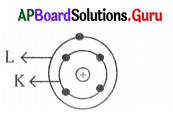A) Number of electrons in K – shell
B) Number of shells
C) Nucleus has V charge
D) No defect
A) Number of electrons in K – shell

Question 87.
For electron distribution 2, 8, 8, the atomic diagram is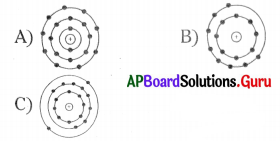D) None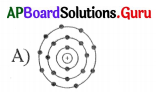Question 88.
Dallons atomic model is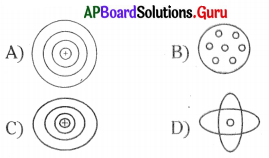Question 89.
Joseph John Thomson is appreciable because, he found
A) first atom
B) electron
C) proton
D) above all
B) electron

Question 90.
Rutherford is appreciable, because he found that
A) Nucleus in the atom
B) Atom has empty space in it
C) Nucleus has a positive charge
D) Above all
D) Above all

Question 91.
Which isotope is very useful to treat goitre ?
A) Iodine
B) Sodium
C) Uranium
D) None
A) Iodine

Question 92.
Which of the following isotope uses for treatment of Cancer ?
A) Iodine
B) Sodium
C) Cobalt
D) None
C) Cobalt

Question 93.
Isotopes are not used in this system.
A) Solving chemical and medical mysteries
B) Investigating the steps of a chemical reaction
C) Medical treatment
D) To pass electricity
D) To pass electricity

Question 94.
Carbon dating is related to
A) carbon isotope $$\left({ }_{6}^{14} \mathrm{C}\right)$$
B) fossils
C) A and B
D) neither A nor B
C) A and BQuestion 95.
Neutrons in gfi $${ }_{8}^{16} \mathrm{O}$$ are
A) 8
B) 16
C) 23
D) Zero
A) 8

Question 96.
Which of the following is a correct electronic configuration of sodium ?
A) 2, 8
B) 8, 2, 1
C)2, 1, 8
D) 2, 8, 1
D) 2, 8, 1

Question 97.
$${ }_{6}^{14} \mathrm{C}$$ isotope is used to find
A) age of fossils
B) nature of genes
C) medical treatment
D) above all
A) age of fossils

Question 98.
The combining capacity of atoms of an element with the atoms of other element is called its valency. Based on valency the odd one is
A) Hydrogen
B) Chlorine
C) Helium
D) Oxygen
C) Helium

Question 99.
Based on scientists and their inventions match Set – I with Set – II:

 Set – I Set – II P. Bohr-Bury x. Existence of a nucleus Q. Thompson y. Maximum no. of electrons in a shell R. Rutherford z. Subatomic particle

A) P – y, Q – z, R – x
B) P – y, Q – x, R – z
C) P – x, Q – y, R – z
D) P – z, Q – x, R – y
A) P – y, Q – z, R – x

Question 100.
Isotopes are used in the laboratory to investigate the steps of chemical reactions and medical mysteries. An isotope which is used in the treatment of Goitre is
A) Iodine isotope
B) Carbon isotope
C) Cobalt isotope
D) Uranium isotope
A) Iodine isotope

Question 101.
Arrangement of electrons in an atom with atomic number (Z) = 5 and valency = 3is
A) 2, 3
B) 1, 1,3
C)2, 1, 2
D) 2, 2, 1
A) 2, 3Question 102.
α-partides scattering experiment helped to detect the existence of the nucleus. The main observation of this experiment is
A) All the alpha particles got deflected.
B) Few no. of alpha particles got deflected.
C) Alpha particles don’t passed . through the gold foil.
D) All the alpha particles passed through the gold foil.
B) Few no. of alpha particles got deflected.

Question 103.
α-particles scattering experiment confirms
i) Nucleus is positively charged.
ii) Nucleus contains electrons.
iii) Electrons revolve around the nucleus.
iv) Most of the space inside the atom is empty.
A) (ii) only
B) (i), (iii) and (iv)
C) (iv) only
D) (i) and (iv)
D) (i) and (iv)

Question 104.

 Shell Name The maximum no. of electrons in a shell K 2 L 8 M X N 32

In the above table ‘x’ can be replaced by
A) 28
B) 30
C) 18
D) 8
C) 18

Question 105.
The standard notation of an atom is $${ }_{\mathbf{Z}}^{\mathrm{A}} \mathbf{X}$$. The option which shows oxygen atom is
A) $${ }_{8}^{16} \mathrm{O}$$
B) $${ }_{16}^{6} \mathrm{O}$$
C) $${ }_{16}^{8} \mathrm{O}$$
D) $${ }_{6}^{16} \mathrm{O}$$
$${ }_{8}^{16} \mathrm{O}$$

Question 106.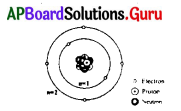The distribution of electrons in the above diagram represents the element
A) Neon
B) Nitrogen
C) Carbon
D) Oxygen
C) Carbon

Question 107.
In the diagram regarding question number (9) the symbol ‘+’ represent
A) Protons
B) Neutrons
C) Protons and Neutrons
D) Electrons
A) Protons

Question 108.
The sum of no. of protons and no. of neutrons is called mass number. The no. of neutrons present in the sodium atom whose atomic number is
A) 11
B) 12
C) 34
D) 23
B) 12Question 109.
In atoms electrons are distributed in different shells. The correct order of distribution of 12 electrons in Magnesium is
A) 2, 8, 2
B) 2, 2, 2, 6
C) 2, 2, 8
D) 2, 2, 6, 2
A) 2, 8, 2

Question 110.
Which metal was used in Rutherford alpha scattering experiment ?
A) Silver
B) Aluminium
C) Gold
D) Platinum
C) Gold

Question 111.
The maximum electrons present in Mshell
A) 2
B) 8
C) 18
D) 32
C) 18

Question 112.
$${ }_{6}^{12} \mathrm{C} \quad{ }_{6}^{13} \mathrm{C} \quad{ }_{6}^{14} \mathrm{C}$$
Observe the carbon isotopes given above and answer the question. What are the neutron numbers of the above isotopes respectively ?
A) 6, 7, 8
B) 6, 6, 6
C) 18, 19, 20
D) 8, 6, 7
A) 6, 7, 8

Question 113.
There are 3 isotopes of carbon. Carbon-12, Carbon-13 and Carbon-14. The most abundant carbon isotope is carbon – 12.
What is the average atomic mass of carbon ?
A) 12.01
B) 14.0
C) 16.0
D) 35.5
A) 12.01

Question 114.
The property of having 8 electrons in the outer most orbit is called
A) Atomicity
B) Valency
C) Octet
D) Inertness
C) OctetQuestion 115.
The number of outermost electrons present in Na+ ion is
A) 8
B) 11
C)10
D) 2
A) 8

Question 116.
If we use iodised salt in our daily we can be prevented by the disease
A) Cancer
B) Goitre
C) Skin disease-Eezima
D) Ulcer
B) Goitre

Question 117.
Goitre – Isotope of iodine :: Cancer : ………………….
A) Isotope of Uranium
B) Isotope of Carbon
C) Isotope of Cobalt
D) Isotope of Chlorine
C) Isotope of Cobalt

Question 118.
In Rutherford’s gold foil experiment, the emitted particles/rays
A) Beta particle
B) Gamma particle
C) X-ray particle
D) Alpha particle
D) Alpha particle

Question 119.
The arrangement of electrons in Oxygen is
A) 2, 2, 2
B) 2, 2, 4
C) 2, 2, 6
D) 2, 4, 2
B) 2, 2, 4

Question 120.
According to which rule, there should be two electrons in the innermost shell
A) Bohr
B) Thomson
C) Bohr-Bury
D) Rutherford
C) Bohr-BuryQuestion 121.
Match the following.

 Set – I Set – II P) Atomic number (Z) S) No. of protons Q) Atomic mass number T) A – Z R) Number of neutrons (n) U) No. of protons + no.of neutrons.

A) P – U, Q – S, R -T
B) P – T, Q – S, R – U
C) P – S, Q -T, R – U
D) P – S, Q – U, R – T